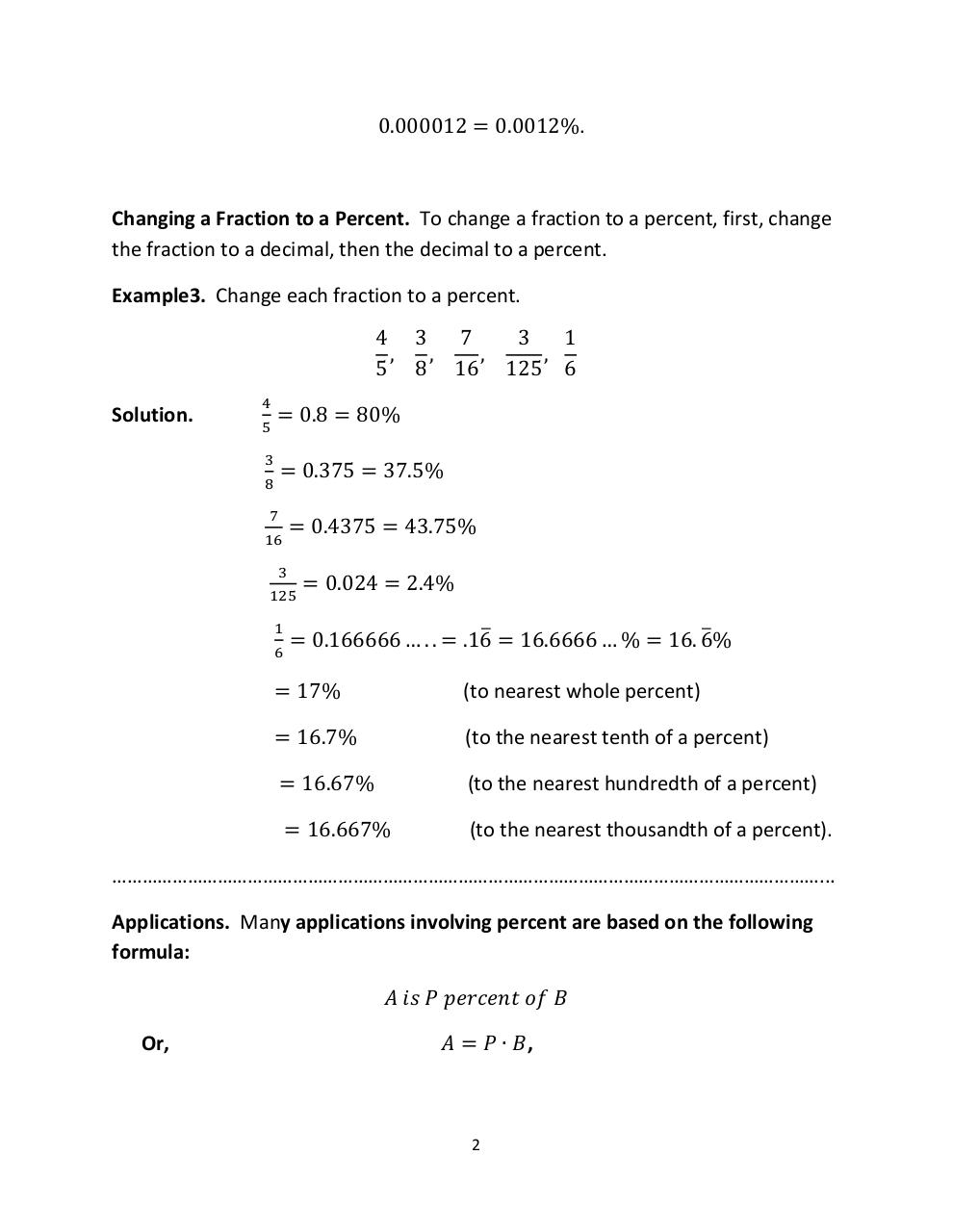# PDF Archive

Easily share your PDF documents with your contacts, on the Web and Social Networks.

## MAT143Sc8.1pdf.pdfPage 1 2 3 4 5

#### Text preview

0.000012 = 0.0012%.

Changing a Fraction to a Percent. To change a fraction to a percent, first, change
the fraction to a decimal, then the decimal to a percent.
Example3. Change each fraction to a percent.
4 3
7
3
1
,
,
,
,
5 8 16 125 6
Solution.

4
5

= 0.8 = 80%

3

= 0.375 = 37.5%

8
7

= 0.4375 = 43.75%

16
3

125
1
6

= 0.024 = 2.4%

= 0.166666 … . . = .16̅ = 16.6666 … % = 16. 6̅%

= 17%

(to nearest whole percent)

= 16.7%

(to the nearest tenth of a percent)

= 16.67%

(to the nearest hundredth of a percent)

= 16.667%

(to the nearest thousandth of a percent).

……………………………………………………………………………………………………………………………...
Applications. Many applications involving percent are based on the following
formula:
𝐴 𝑖𝑠 𝑃 𝑝𝑒𝑟𝑐𝑒𝑛𝑡 𝑜𝑓 𝐵
Or,

𝐴 = 𝑃 ∙ 𝐵,

2